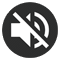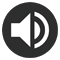# 12 Times table Chart - Printable PDF

3 Reviews

## Print the 12 Times table Chart

A pretty 12 Times table chart in A4 format (PDF) that will help you learn your 12 times table. Thanks to its colored numbers, it will make it easier for you to memorize the multiplication results.

Print for free this 12 times table and don't forget to take a look at the time-saving trick for the 12 times table. Then, practice online this times table. It will help you memorize the table even faster.

This chart is also available in other colors or in black and white. Feel free to look at all the templates we propose below and choose your favorite one!
In a Multiplication Chart, the results are displayed in a table (with columns and rows), whereas in a Times table Chart they are displayed as a list of increasing multiplications.

Here the list starts at 12x1 and ends at 12x12. One way to memorize your table is to recite it aloud in this way:

12 times 1... 12,  12 times 2... 24,  12 times 3... 36,  12 times 4... 48,  12 times 5... 60,  12 times 6... 72,  12 times 7... 84,  12 times 8... 96,  12 times 9... 108,  12 times 10... 120,  12 times 11... 132,  12 times 12... 144

This way of learning using auditory memory is effective for many children.

Print the 12 Times table Chart (PDF)

If you plan to print the other times tables, we advise you to choose a different color for each multiplication table from 1 to 12. To save time, you will find, in our All Times tables section, PDFs including all the tables colored like a rainbow.

## Our other 12 Times table PDF

Thanks to the many colors available, you will undoubtedly find your favorite chart! Our black and white 12 Times table charts will be perfect if you prefer sobriety, if you want to save ink or if you prefer to color your times table yourself using your pencils or markers. Feel free to click on the images to preview the PDF or use the "PRINT" button below the image to download the chart of your choice.

12 Times table Chart
Black & white
Print
12 Times table Chart
Handwritten numbers
Print
12 Times table Chart
Ink saving
Print
12 Times table Chart
Grey
Print
12 Times table Chart
Dark green
Print
12 Times table Chart
Green
Print
12 Times table Chart
Light green
Print
12 Times table Chart
Turquoise
Print
12 Times table Chart
Blue
Print
12 Times table Chart
Dark blue
Print
12 Times table Chart
Purple
Print
12 Times table Chart
Pink
Print
12 Times table Chart
Light pink
Print

## Trick for the 12 times table

This table may seem difficult, but you'll see that with our trick the table of 12 is child's play! You'll be able to quickly find the result of a multiplication up to 12 x 12 and even beyond, without needing your calculator!

You need to know that when you multiply a number by 12, it's the same thing as if you multiply it by 10 on one side, by 2 on the other and then add the 2 results together.
Let's take the example of the multiplication 12 x 4:
1- Decompose the multiplication:
12 x 4 = 10 x 4 + 2 x 4
12 x 4 = 40 + 8 = 48

### The results of the 12 times table

• 12 x 1 = 12  (see the 1 times table)
• 12 x 2 = 24  (= 10 x 2 + 2 x 2)
• 12 x 3 = 36  (= 10 x 3 + 2 x 3)
• 12 x 4 = 48  (= 10 x 4 + 2 x 4)
• 12 x 5 = 60  (= 10 x 5 + 2 x 5)
• 12 x 6 = 72  (= 10 x 6 + 2 x 6)
• 12 x 7 = 84  (= 10 x 7 + 2 x 7)
• 12 x 8 = 96  (= 10 x 8 + 2 x 8)
• 12 x 9 = 108  (= 10 x 9 + 2 x 9)
• 12 x 10 = 120  (see the 10 times table)
• 12 x 11 = 132  (see the 11 times table)
• 12 x 12 = 144  (= 10 x 12 + 2 x 12)
• ... (this trick also works for multiplications higher than 12 x 12)

## Practice the 12 times table

In this exercise, you can choose between several settings, so it's up to you to select the ones that suit you best. Here are the available options:

• Number color: By choosing the colorful numbers option, the color code of the table of 12 (red) is applied. This is the same color code as the printable 12 times table chart at the top of the page. The use of color helps memorization, so we highly recommend the colorful mode!
• Number format: you can choose a specific font for the numbers. There are standard fonts, but also school fonts. Select the one that's closest to the one used at school.
• Game mode: random or increasing.

If you like this exercise, you can also practice the other tables in the All times tables section.

USEFUL !
You can see your more common mistakes below the settings.NUMBERS :
NUMBER FORMAT :
MULTIPLICATIONS :
GAME MODE :

### Your most common mistakes :

You will find here the list of your most common mistakes

### A chart with all the times tables (2 PDF to cut and assemble)

A pretty chart with all the times tables to print in A4 format (PDF). We have assigned a different color code for each times table, with a nice color gradient. The colors will help you memorize all your multiplication tables from the 1 to the 12 times table (other colors are available for this resource).

See the Chart